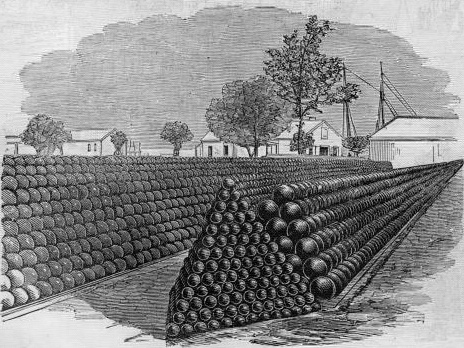### See a Proof of Kepler’s Conjecture

In 1611, Kepler proposed that the closest sphere packing has a maximum density of pi/(3sqrt(2)) approx 74%), this became known as Kepler conjecture. Sure Buckminster Fuller (1975) claimed to have a proof, but it was really a description of face-centered cubic packing, not a proof of its optimality, which was produced by Sloane in 1998. But lets back up a bit and start with…A quick, simple power point presentation about:

Kepler’s Conjecture about sphere packing – by Alina

Once you are happy with the problem, lets see how it was dealt with in

A 21 Page proof of the Conjecture by Students of Math at Princeton University

Authored by Thomas Hales, Mark Adams, Gertrud Bauer, Dang Tat Dat, John Harrison, Hoang Le Truong, Cezary Kaliszyk, Victor Magron, Sean Mclaughlin, Nguyen Tat Thang, nguyen Quang Truong, Tobias Nipkow, Steven Obua, Joseph Pleso, Jason Rute, alexey Solovyev, Ta Thi Hoai An, Tran Nam Trung, Trieu Thi Diep, Josef Urban, VuKhac Ky, Roland Zumkeller.

Or look deeper, and check out the full…

121 Page Proof By Thomas C. Hales of Princeton University

In this pdf file The Kepler conjecture is defined as: No packing of congruent balls in Euclidean three space has density greater than that of the face-centered cubic packing. This density is π/√18 ≈ 0.74.

The proof of this result is presented in this paper. Here, we describe the top-level outline of the proof and give references to the sources of the details of the proof.

An expository account of the proof is contained in [Hal00]. A general reference on sphere packings is [CS98]. A general discussion of the computer algorithms that are used in the proof can be found in [Hal03]. Some speculations on the structure of a second-generation proof can be found in [Hal01].
Details of computer calculations can be found on the internet at [Hal05].

The current paper presents an abridged form of the proof. The full proof appears in [Hal06a]. Samuel P. Ferguson has made important contributions to this proof. His University of Michigan thesis gives the proof of a difficult part of the proof [Fer97].

By a packing, we mean an arrangement of congruent balls that are non-overlapping in the sense that the interiors of the balls are pairwise disjoint. Con-1068 THOMAS C. HALES sider a packing of congruent balls in Euclidean three space. There is no harm in assuming that all the balls have unit radius. The density of a packing does not decrease when balls are added to the packing. Thus, to answer a question about the greatest possible density we may add nonoverlapping balls until there
is no room to add further balls. Such a packing will be said to be saturated.